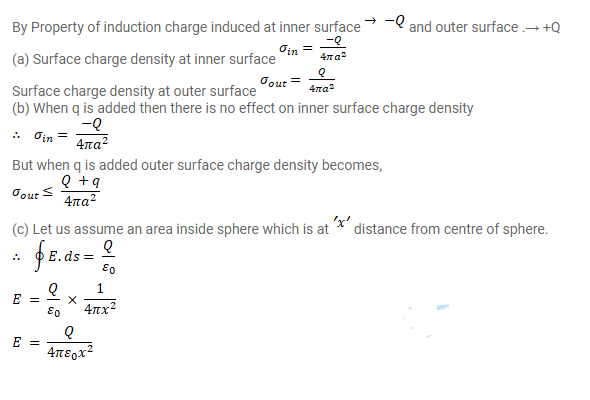# A charge Q is placed at the centre of an uncharged,`
Question:

A charge $\mathrm{Q}$ is placed at the centre of an uncharged, hollow metallic sphere of radius a'

(a) Find the surface charge density on the inner surface and on the outer surface.

(b) If a charge $q$ is put on the sphere, what would be the surface charge densities on the inner and the outer surfaces? (c) Find the electric field inside the sphere at a distance $x$ from the centre in the situations (a) and (b).

Solution: